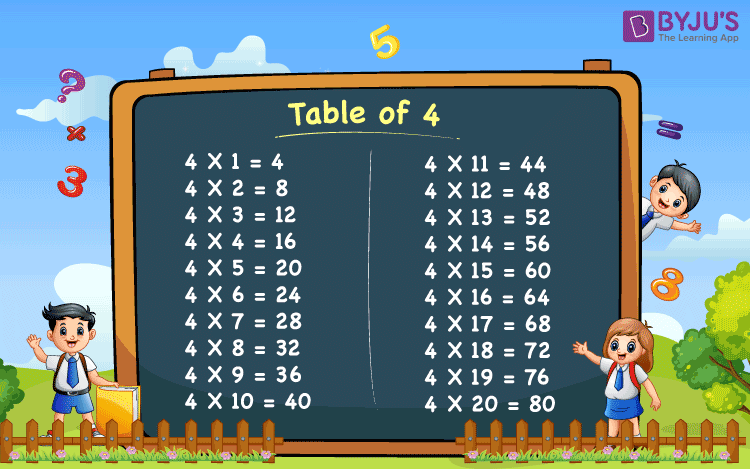# Table of 4

Table of 4 is provided here. It is essential for the students to memorise the table which can help them in fast maths calculation. Learning table helps students to save a lot of time, especially in the time-based examination. Students can find table of 4 up to 20 times that needs to be memorised for fast calculation and solving the problems.

## Table of 4 Chart## Multiplication Table of 4

 4 x 1 = 4 4 x 2 = 8 4 x 3 = 12 4 x 4 = 16 4 x 5 = 20 4 x 6 = 24 4 x 7 = 28 4 x 8 = 32 4 x 9 = 36 4 x 10 = 40 4 x 11 = 44 4 x 12 = 48 4 x 13 = 52 4 x 14 = 56 4 x 15 = 60 4 x 16 = 64 4 x 17 = 68 4 x 18 = 72 4 x 19 = 76 4 x 20 = 80

Get More Maths Tables:-

Test your Knowledge on Table of 4